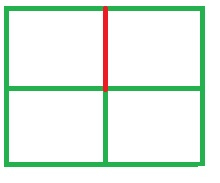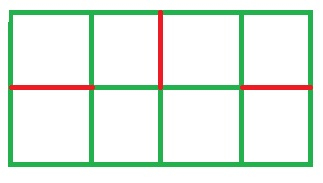# Minimum Cuts can be made in the Chessboard such that it is not divided into 2 parts in C++

## Concept

Given A x B Chessboard, the task is to calculate the Maximum numbers of cuts that we can build in the Chessboard so that the Chessboard is not divided into 2 parts.

Examples

The examples are given below −

Input

A = 2, B = 4

Output

Number of maximum cuts = 3

Input

A = 2, B = 2

Output

Number of maximum cuts = 1

## Method

• For A = 2, B = 2 ,we can only build 1 cut (mark in red). If we build 1 more cut then the chessboard will divide into 2 pieces• For A = 2, B = 4 ,we can makes 3 cuts (marks in red). If we build 1 more cut then the chessboard will divide into 2 pieces.As a result of this, it can be observed that no. of cuts = (A-1) * (B-1).

## Example

Live Demo

//This is C++ implementation of above approach
#include <bits/stdc++.h>
using namespace std;
// function that calculates the maximum no. of cuts
int numberOfCuts1(int A, int B){
int result1 = 0;
result1 = (A - 1) * (B - 1);
return result1;
}
// Driver Code
int main(){
int A = 4, B = 4;
// Calling function.
int Cuts = numberOfCuts1(A, B);
cout << "Maximum cuts = " << Cuts;
return 0;
}

## Output

Maximum cuts = 9

Updated on: 23-Jul-2020

109 Views